Updating search results...

# 14 Results

View
Selected filters:
• fraction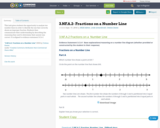Conditional Remix & Share Permitted
CC BY-NC-SA
Rating
0.0 stars

This task gives students the opportunity to analyze two number lines in order to identify the one that correctly shows an improper fraction. Students then communicate their understanding by describing the reasoning they used to determine their answer was correct. It is aligned to evidence statement 3.C.6-1

Subject:
Education
Mathematics
Material Type:
Activity/Lab
Assessment
Homework/Assignment
09/17/2018Only Sharing Permitted
CC BY-NC-ND
Rating
0.0 stars

Subject:
Mathematics
Material Type:
Activity/Lab
Assessment
Homework/Assignment
08/30/2018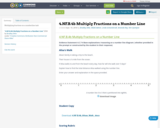Conditional Remix & Share Permitted
CC BY-NC-SA
Rating
0.0 stars

Multiplying fractions on a numberline task

Subject:
Mathematics
Material Type:
Assessment
09/14/2018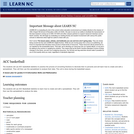Conditional Remix & Share Permitted
CC BY-NC-SA
Rating
0.0 stars

The students will use ACC basketball statistics to practice the process of converting fractions to decimals then to percents and will learn how to create and edit a spreadsheet. They will then use this spreadsheet to analyze their data. This unit is done during the basketball season which takes approximately 15 weeks from the middle of November to the middle of March. Teachers must have Clarisworks to open the sample spreadsheet in the lesson, but may recreate it in another spreadsheet program.

Subject:
Mathematics
Statistics and Probability
Material Type:
Lesson Plan
Provider:
University of North Carolina at Chapel Hill School of Education
Provider Set:
LEARN NC Lesson Plans
Author:
Susan Dougherty
07/14/2000Only Sharing Permitted
CC BY-NC-ND
Rating
0.0 stars

BPCC Open Campus - Math 097: Basic Mathematics is a review of basic mathematics skills. Here's what's covered: -fundamental numeral operations of addition, subtraction, multiplication division of whole numbers, fractions, and decimals -ratio and proportion -percent -systems of measurement -an introduction to geometry NOTE: Open Campus courses are non-credit reviews and tutorials and cannot be used to satisfy requirements in any curriculum at BPCC.

Subject:
Education
Mathematics
Material Type:
Assessment
Full Course
Lecture
Lesson
Unit of Study
10/11/2017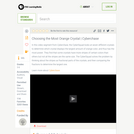Educational Use
Rating
0.0 stars

In this video segment from Cyberchase, the CyberSquad must locate a crystal with the highest fraction of orange color.

Subject:
Mathematics
Material Type:
Lecture
Provider:
PBS LearningMedia
Provider Set:
PBS Learning Media: Multimedia Resources for the Classroom and Professional Development
Author:
U.S. Department of Education
WNET
07/08/2008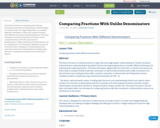Unrestricted Use
CC BY
Rating
0.0 stars

This lesson focuses on comparing and ordering fractions in ways that encourage deeper understanding of’ ‘number sense’ by supporting learners to consider different techniques to order and compare fractions with different numerators and denominators. The three techniques covered in this lesson are those used to compare fractions with like numerators or denominators, unlike numerators or denominators and by comparing to a 1/2 benchmark. Emphasis are placed on the two latter techniques. Activities and practice exercises involve real-world problems including sales discounts, cooking measurements and school score reports.

Subject:
Mathematics
Material Type:
Homework/Assignment
Lesson Plan
12/13/2016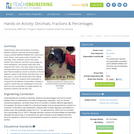Educational Use
Rating
0.0 stars

Students learn about and practice converting between fractions, decimals and percentages. Using a LEGO® MINDSTORMS® NXT robot and a touch sensor, each group inputs a fraction of its choosing. Team members convert this same fraction into a decimal, and then a percentage via hand calculations, and double check their work using the NXT robot. Then they observe the robot moving forward and record that distance. Students learn that the distance moved is a fraction of the full distance, based on the fraction that they input, so if they input ½, the robot moves half of the original distance. From this, students work backwards to compute the full distance. Groups then compete in a game in which they are challenged to move the robot as close as possible to a target distance by inputting a fraction into the NXT bot.

Subject:
Applied Science
Engineering
Mathematics
Technology
Material Type:
Activity/Lab
Provider:
TeachEngineering
Provider Set:
TeachEngineering
Author:
Javed Narain
09/18/2014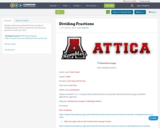Conditional Remix & Share Permitted
CC BY-NC
Rating
0.0 stars

Students will review and practice the concepts of dividing fractions. This is a great tool to use for extra practice or review for a test.

Subject:
Mathematics
Material Type:
Activity/Lab
06/09/2017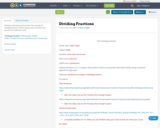Conditional Remix & Share Permitted
CC BY-NC
Rating
0.0 stars

Students will review and practice the concepts of dividing fractions. This is a great tool to use for extra practice or review for a test.

Subject:
Mathematics
Material Type:
Activity/Lab
10/16/2019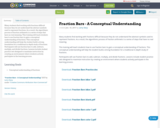Conditional Remix & Share Permitted
CC BY-NC
Rating
0.0 stars

Many students find working with fractions difficult because they do not understand the abstract symbols used to represent fractions. As a result, the algorithmic process of fraction arithmetic is a series of steps that have no real meaning.

This training will teach students how to use fraction bars to gain a conceptual understanding of fractions. This conceptual understanding will help the student build a strong foundation for a traditional in-depth study of fractions.

Participants will use fraction bars to add, subtract, multiply, and divide fractions. Lessons include student practice sets designed to maximize instruction by creating an environment where students actively participate in the learning process.

Subject:
Mathematics
Material Type:
Lesson
01/18/2017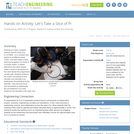Educational Use
Rating
0.0 stars

Working as a team, students discover that the value of pi (3.1415926...) is a constant and applies to all different sized circles. The team builds a basic robot and programs it to travel in a circular motion. A marker attached to the robot chassis draws a circle on the ground as the robot travels the programmed circular path. Students measure the circle's circumference and diameter and calculate pi by dividing the circumference by the diameter. They discover the pi and circumference relationship; the circumference of a circle divided by the diameter is the value of pi.

Subject:
Applied Science
Computing and Information
Engineering
Mathematics
Technology
Material Type:
Activity/Lab
Provider:
TeachEngineering
Provider Set:
TeachEngineering
Author:
Carole Chen
Michael Hernandez
09/18/2014Conditional Remix & Share Permitted
CC BY-NC
Rating
0.0 stars

In this lesson you will learn how to convert between decimal, percent and fraction; calculate percent of change; and apply percents to real-world situations. &nbsp;You will be able to discuss the importance of writing numbers in different formats; how to determine a percent increase or decrease; and how percents are an integral part of daily living.StandardsCC.2.1.HS.F.2Apply properties of rational and irrational numbers to solve real world or mathematical problems.MP.1. Make sense of problems and persevere in solving them.MP.4. Model with mathematics.MP.5. Use appropriate tools strategically.

Subject:
Algebra
Mathematics
Material Type:
Lesson Plan
Author:
Tracy Rains
04/05/2018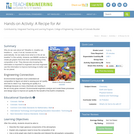Educational Use
Rating
0.0 stars

Why do we care about air? Breathe in, breathe out, breathe in... most, if not all, humans do this automatically. Do we really know what is in the air we breathe? In this activity, students use M&M(TM) candies to create pie graphs that show their understanding of the composition of air. They discuss why knowing this information is important to engineers and how engineers use this information to improve technology to better care for our planet.

Subject:
Applied Science
Atmospheric Science
Engineering
Physical Science
Material Type:
Activity/Lab
Provider:
TeachEngineering
Provider Set:
TeachEngineering
Author:
Amy Kolenbrander
Daria Kotys-Schwartz
Denise W. Carlson
Janet Yowell
Malinda Schaefer Zarske
Natalie Mach